# Category Archives: MathsFiles.com Resources

Posts about Files and other Resources available on MathsFiles.com

# The Offset Function and Dynamic Named Ranges in Excel

Understanding the Offset function and its use in creating dynamic named ranges opens up some very powerful techniques in Excel.
One such use is in creating a dynamic chart which updates automatically when its linked data is changed.
Although this can be more easily done in Excel 2007 onwards by using Tables the dynamic named range method is more powerful and has a wider range of uses.I have used these techniques extensively in my Excel work.

This excel file available in the Excel techniques section of www.mathsfiles.com/Excel_Files shows how the Offset function works and how it can be used to create a simple dynamic graph.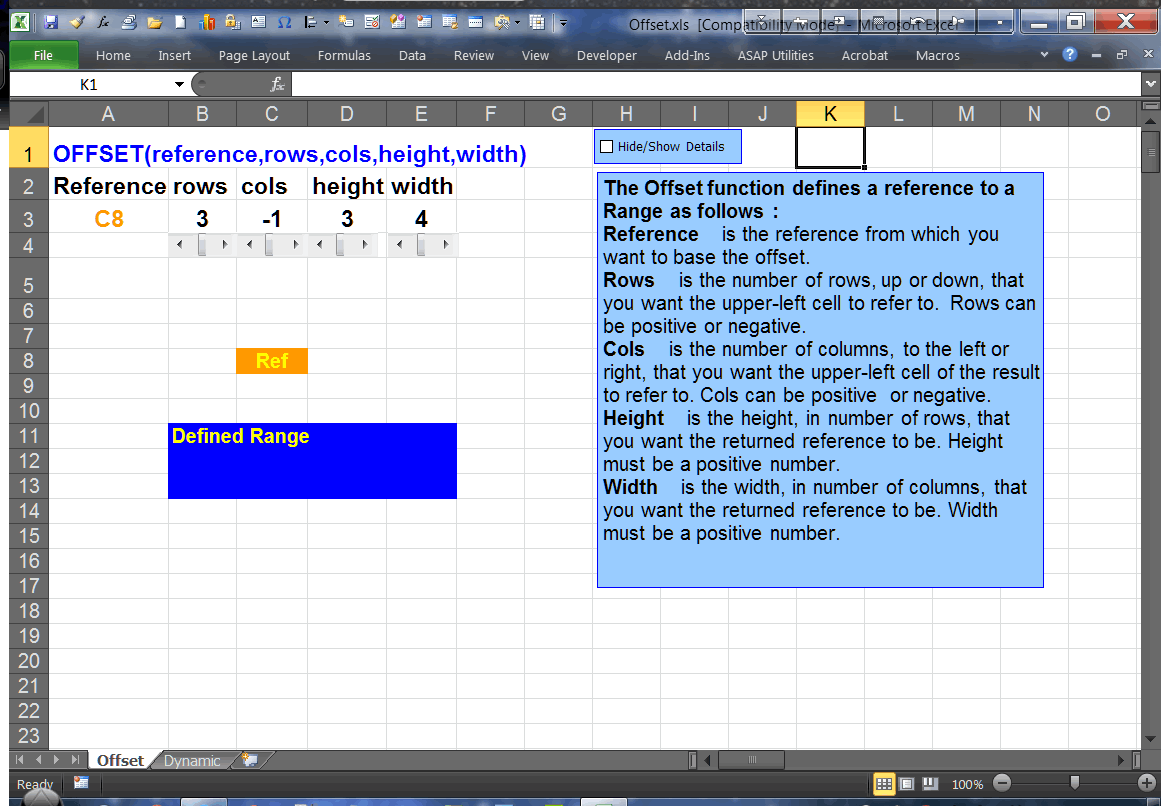Offset function screen shot

Direct link:
Offset Function Excel file

You can also watch a Demo which shows in more detail how to set up a dynamic named range and use it to create a dynamic graph.

Watch the Demo (based on Excel 2007) or

Watch the Demo (based on Excel 2003)

# Creating Scroll Bars in Excel

I remember the first time I saw a scroll bar in Excel. ‘Wow!’ I thought, ‘I can think of so many things I could do with that’.

Scroll bars can be used to control the value of a cell so that we can see the effect of changing its value on a graph or calculation. They are great for creating dynamic demonstrations or explorations.

However the basic scroll bar that Excel creates for you is a bit limited and needs some work to get it to do everything we might want from it.

This excel file available in the Excel techniques section of www.mathsfiles.com/Excel_Files shows how to create and configure scroll bars to achieve our goals. We start with the most basic and work through to a sophisticated macro controlled version.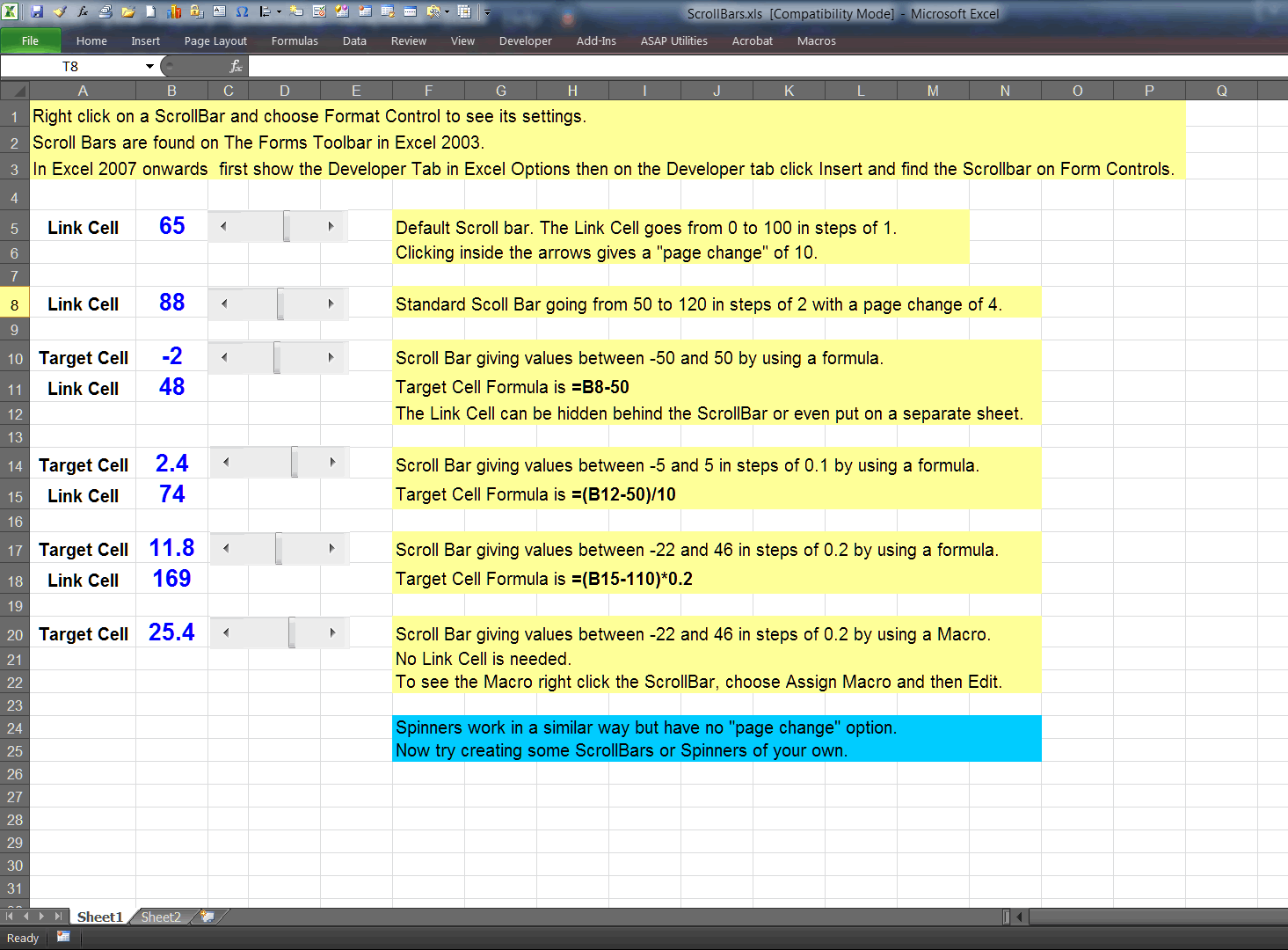Scroll Bars in Excel

Direct link:
Scroll Bars Excel file

# Setting Up Excel for Advanced Work

This covers Customizing the Quick Access Toolbar and enabling the Developer Tab for Excel 2007 onwards:

If you want to work with Macros and other Advanced features in Excel you need to show the Developer Tab and it’s also a good idea to customize the Quick Access Toolbar to give you easy access to the key tools.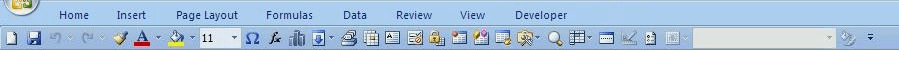Excel after customization

There are two files on www.mathsfiles.com/Excel_Files in the techniques section that show how to do this.

Direct links:
Instructions pdf file

Watch a Demo (based on Excel 2007)
Note: The process is much the same for later versions of Excel but the way to show the Developer Tab is found by going to Excel Options and then the section for Customizing the Ribbon. Just  check the box for the Developer Tab.

# What is an Excel Macro?

An Introduction to Recording and Modifying an Excel Macro.

On a basic level one can use the Macro recorder to record some actions in Excel without looking at the code generated.
The macro can then be attached to a button or run with a keyboard shortcut to replay those actions.
At the next level one can look at the generated code in the Visual Basic Editor and start to understand what it does and begin to be able to tweak it to achieve something a bit more sophisticated.
As one learns more and with the aid of internet forums and tutorials, it becomes possible to write code oneself and/or to use and adapt other people’s code. Looping structures and other computer programming forms can be used.
There are also special Excel Macros that are triggered by events such as when the worksheet recalculates. These can be used to create impressive effects.

I have created a demo screen recording which shows how we can begin to use the Macro Recorder to learn about and modify VBA code.

You can find the demo on the Excel techniques section of the Excel page at MathsFiles.com or click on this direct link :
Macro Recorder demo.

# 3D Matrices: Multiplication, Determinant and Inverse

There are three Excel files available for download at www.mathsfiles.com/Excel_Files under A/AS Pure Files that can help with explaining these processes in a visual and interactive may.
Sometimes a picture is worth a thousand words and I find that once students see these operations explained in this graphic way they “get it” and remember it in a quick and natural way.
The files use Macros so that if you click on an element the highlighting will change dynamically to show the how the calculation is done.
The entries in the Matrices can also be changed to generate new examples which is useful if the teacher wants to set a question and then later use the files to get the students to check their working.

Here are some screenshots, click to enlarge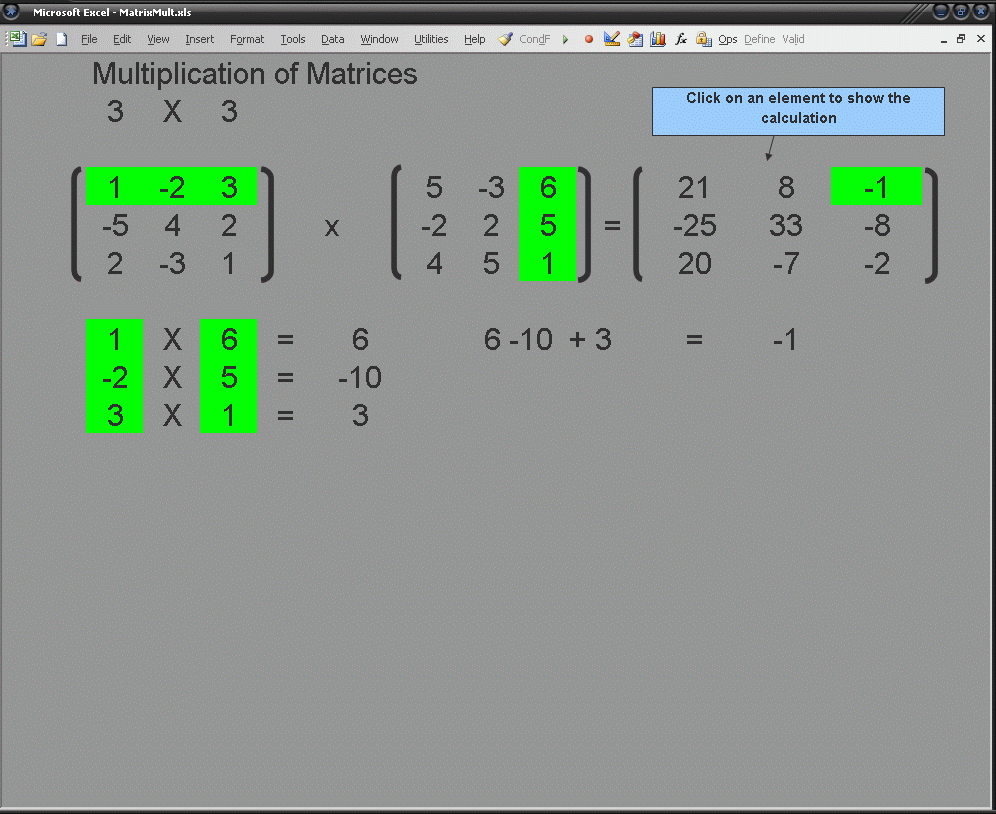Matrix MultiplicationMatrix Determinant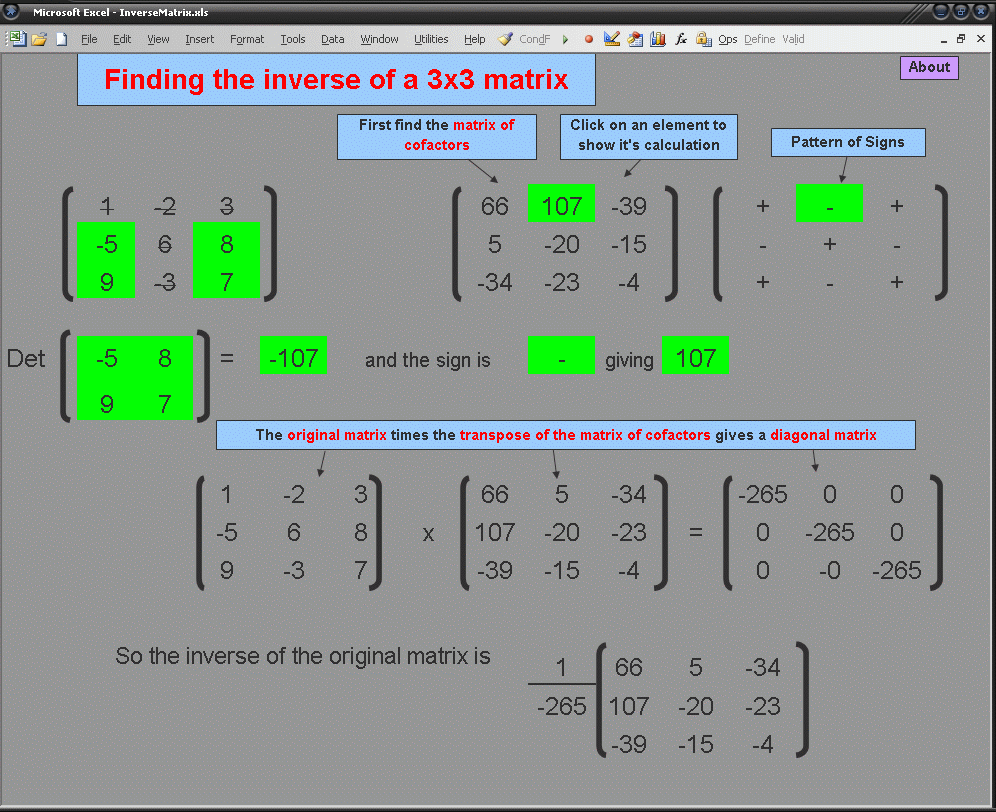Matrix Inverse

# Normal Distribution Trainer

There are two files on MathFiles.com which aim to teach a systematic and efficient approach to solving basic Normal distribution problems.
The method I use is summarised in Normal Distribution Problems pdf
This method can then be demonstrated and practiced using the Normal Trainer Excel file.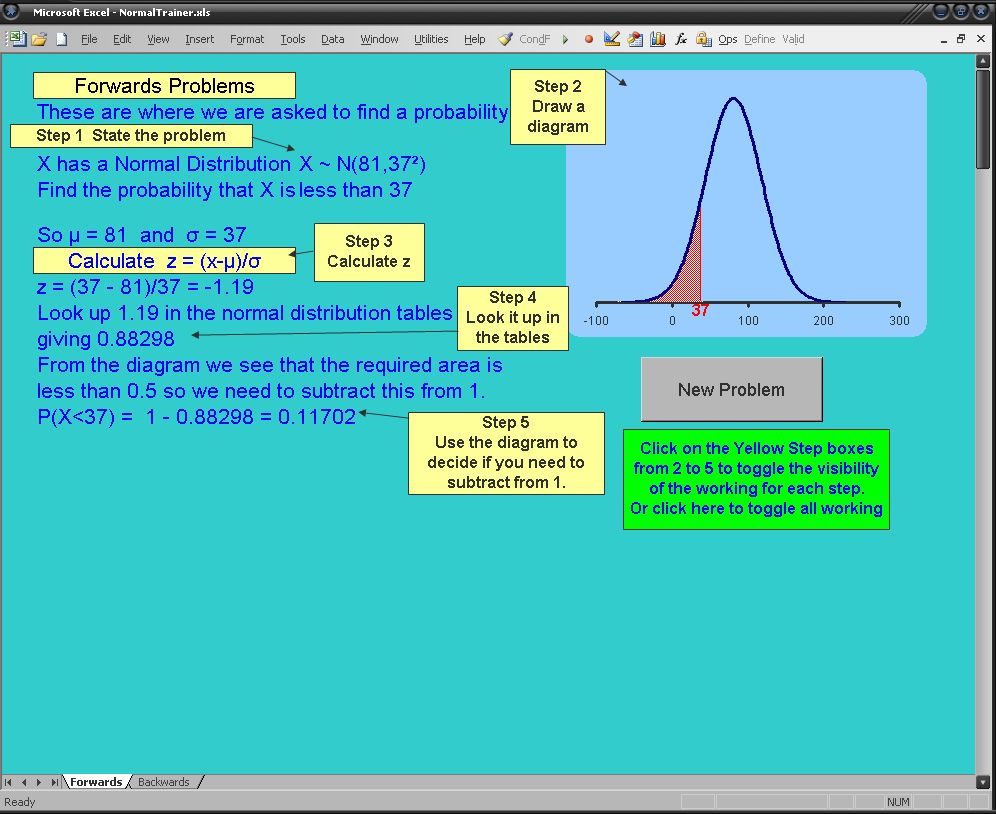The Normal Trainer Excel File

First I emphasise the distinction between ‘Forwards’ and ‘Backwards’ problems: A ‘Forwards’ problem is where we are given information and asked to find a probability; a ‘Backwards’ problem is where we are given a probability and asked to find something else: either the mean the standard deviation or a value of X.
For ‘Forwards’ problems the key strategy is to draw a diagram showing the area of the Normal distribution graph which represents the probability we are trying to find. If this area is seen to be greater than 0.5 we simply need to calculate and then look up the z-value in tables and write down the corresponding probability.
If the area is seen to be less than 0.5 we calculate and look up the z-value in tables and take the corresponding probability away from 1. The important thing here is to realise that this strategy will work independently of whether z is positive or negative and hence it simplifies the process considerably.

For ‘Backwards’ problems the key step is to draw a Normal distribution diagram showing the area representing the probability, p, we are given in the question and also the area representing the probability of the opposite event (1-p). We then reverse look up the largest of these two probabilities in the Normal distribution tables to find the z-value and determine if it is positive or negative by using the diagram: if z is on the left it’s negative, if it’s on the right it’s positive. Finally we put all our information into the standardization formula and solve for the unknown.